Induced eddy currents in a steel sheet

Woo, another great animation!

In case you don’t immediately see (huh, weird) what’s happening, here’s the short story:

### Animation explained

The rectangle you see is the cross section of an electric steel sheet. The shorther edge is along the sheet thickness, 1 mm in this case. The magnetic flux density is travelling perpendicularly to the cross section, i.e. in and out of your screen. Furthermore, the average flux density (or the total flux, for that matter) is varying sinusoidally at a frequency of 500 Hz.

You see two things in the video. The color scale depicts the magnitude of the flux density. The arrows, on the other hand, are the induced eddy-current density.

##### What happens in Bertotti model

What you really see is, in fact, the phenomenon behind the so-called macroscopic eddy-current loss in iron. The flux density is varying in time, so of course currents are induced in the electrically-conductive iron. These currents oppose the change in flux, and result in power dissipated as heat.

Typically, this effect is considered by a simple, analytically-derived coefficient. To keep things simple, the effect of the induced currents on the flux is more or less neglected.

##### What happens in reality

However, take another look at the video. You can see how the flux gets concentrated near the sheet edges. This is nothing more than the skin effect in action.

Typically in analysis, this effect is ignored and the flux density is assumed uniform along the sheet thickness, while in reality it is anything but. This can generate error by several means.

First of all, the current density can differ from the simple analytical solution, leading to different resistive losses. Secondly, the local flux density differs too, and can reach higher values than expected from the along-the-sheer average. Finally, the repulsive effect of the eddy currents on the flux could mean that a little more phase current is needed to drive the flux.

Luckily, the results are usually nothing catastrophic. However, the effect can still be worth considering in thick sheets such as pole shoes, or sheets subject to high frequencies. Also luckily, the phenomenon can be modelled quite nicely even in 2D analysis. In other words, a colossal 3D simulation with individual sheets meshed is normally not needed.

Lets see how it happens.

### Problem formulation

Anyway, let’s get back to the animation and how it was computed. There’s nothing fancy there, really. It’s just a brute-force simulation, using the standard AV-formulation, using SMEKlib.

However, it is still different from typical 2D problems. You remember, normally the flux is flowing in the plane, while the vector potential and currents are z-directional.

Now, however, the situation is flipped. The flux is perpendicular to the plane, necessitating that the vector potential lies on it. Due to this, we have to solve a vector quantity, rather than a scalar as usually.

Sidenote: it would have been possible to use the T-Omega formulation instead. In that case, we would have solved for a current vector potential and a magnetic scalar potential (instead of magnetic vector and electric scalar, like we do with the AV approach). Both of these would have been essentially scalar for the 2D problem. However, based on a brief look it seemed that some nonstandard matrices would have been needed as the problem is nonlinear. Thus, AV it was.

##### Nedelec elements

As many can probably guess, A was discretized using Nedelec elements, to put it in a really fancy way. What it means is this:

Take a look at this post again. See how the unknown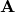is written as a sum of known functions, called shape functions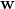. Now, the shape functions simply happen to be vector-valued, and were originally invented by one Mr. Nedelec. Dunno why people use the term Nedelec elements, as the elements are still the same triangles as ever.

Anyways, the good thing about Nedelecs is that they are tangentially continuous everywhere, which happens to be the requirement for the vector potential too. So they fit this problem quite nicely.

##### Back to problem

And finally, despite the name AV formulation, the V part was not really solved. This was thanks to some mathematical hand-wringing. You see, only the gradient of the electric potential V appears in the problem, as it is the gradient that’s driving the currents.

On the other hand, the curl kills gradients, so we can add any gradient field toand still get the same field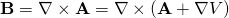.

Now, the only thing left to do is decide that hey let’s just say the V-field is already hidden insideand simply drop it altogether. This is called the reduced vector potential approach, and works nicely when the entire problem domain is conductive. Otherwise, it won’t work at all.

##### Boundary conditions

There’s one more theoretical aspect to consider. As mentioned earlier, the total flux going through the sheet was fixed. However, within the sheet, the flux was allowed to distribute freely, dictated by the induced eddy currents as well as local saturation.

Fixing the total flux like this is by no means a standard constraint. However, it turns out it can still be quite easily enforced.

Let’s begin with the definition of flux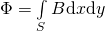.

By plugging in the definition of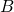by the vector potential, and invoking the Stokes theorem, we get something as simple as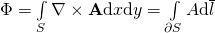.

In other words, the flux is equal to a line integral of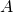around the sheet.

##### Enforcing the flux with Lagrange multipliers

This can then be enforced with Lagrange multipliers, something I have already written about earlier. Nevertheless, let’s take a brief look on them from the physics point of view.

Indeed, it turns out that the single Lagrange multiplier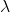corresponds to a thin current layer around the sheet. Similar to what is used commonly in analytical torque computations, that is. The earlier flux equation is then a type of a voltage equation fixing the surface current, in such a way that we get the correct total flux.

The current sheet fixes the tangential component of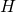on the boundary, and therefore the normal derivative of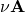. In other words, it corresponds to a Neumann boundary condition, leading to the load vector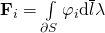for the discrete problem.

On the other hand, the flux constraint can be written as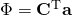where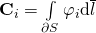,

and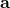is the discretized vector potential.

So, we get the kind of symmetric saddle-point problem we can expect with Lagrangian stuff.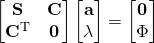.

### Conclusion

When thick laminations (for a specific frequency are used), the induced eddy currents push the flux towards its edges. This leads to local saturation and increased losses. The phenomenon can be approximately modelled in 2D, but the problem formulation is quite different from typical problems. Finally, the average flux density can be fixed with Lagrange multipliers, corresponding to a current sheet.

Check out EMDtool - Electric Motor Design toolbox for Matlab.

Need help with electric motor design or design software? Let's get in touch - satisfaction guaranteed!Induced eddy currents in sheet – SMEKlib example

### 4 thoughts on “Induced eddy currents in sheet – SMEKlib example”

•Chengliu Ai says:

Hi Antti, my name is Chengliu Ai. Now I am an assistant professor in the Institute of Electrical Engineering, Chinese Academy of Sciences. I am excited to see that you realized the FEA in Matlab. I would like to use your Matlab FEA code to compute a 11kW induction motor with skewed rotor. Therefore, I wonder if your 2D Matlab FEA could calculate the skewed effect of the induction motor by using the Multi-Slice FEM. Looking forward to your reply.

1.Antti (admin) says:

Hi Chengliu, and congrats on your assistant prof. position! Sure, I have used a sliced model myself, although haven’t published one yet. So it shouldn’t be any problem at all. We can discuss the details by email.

•Anthony Frias says:

Hello Anti,
I very much like what you have done here. And even more the fact that you make the code available. I see that you have some code to get the result for non linear material as well.
I am trying to use it to get the “macroscopic” B and H. Basically the B and H that you would mesure outside of the specimen if this was an experiment in the real world. B is easy to get. H seems a little more tricky. Then I would like to try to change the excitation wave form.

The ultimate goal is to draw some “dynamic hysteresis” loops and compare it (or try to fit it) to some measured data. I am hoping for some useful results.

There is a threshold in the understanding of the FEM and the code that I have not quite overcome yet though.

1.Antti (admin) says:

Hello Anthony, and thanks for your words!

The dynamic hysteresis thingy is a very interesting topic! I know the loop gets wider at higher frequencies, and suspect it is indeed largely due to in-lamination eddies. But I haven’t had any time to look deeper into it.

For the macroscopic H, I THINK the Lagrange multiplier directly corresponds to the average field strength, times -1. I’ll update the example so that the instantaneous (B,H) points are plotted; it seems to work correctly based on some simple tests at least.